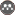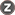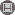BibTeX | RIS | EndNote | Medlars | ProCite | Reference Manager | RefWorks
Send citation to:Oraizi H, Siahkari M J. Analysis and design of asymmetrical multi-section directional coupler with input/output impedance matching. Journal of Iranian Association of Electrical and Electronics Engineers. 2020; 17 (3) :101-107
URL: http://jiaeee.com/article-1-482-en.html
Iran University Science and Technology
Abstract:   (802 Views)

This paper presents the analysis and design of an asymmetrical directional coupler (ADC) with arbitrary source and load impedances. The microstrip-coupled-line structure is analyzed and its impedance matrix is first obtained. Its transmission matrix is then determined. The transmission matrix of multi-section ADC is then obtained using its properties. Finally, the total transmission matrix is converted to the impedance matrix and then its scattering matrix is derived. The method of least squares (MLS) is applied for the design of ADC. An error function is constructed based on the scattering parameters of ADC, which is a function of its geometrical dimensions. The minimization of the error function determines its dimensions (namely widths and lengths of line sections and their spacings) by writing a software in MATLAB using the Genetic Algorithm (GA) and Conjugate Gradient Algorithm (CGM). The HFSS Full Wave Software is applied to finalize the optimum design of the ADC configuration. An example of the ADC is designed, fabricated (on the substrate ROGERS 4003) and measured as the proof of concept. Its coupling coefficient is 6 dB, frequency band is 5.2-6.2 GHz, terminal impedances are 50, 50, 75 and 100 Ohms.